Question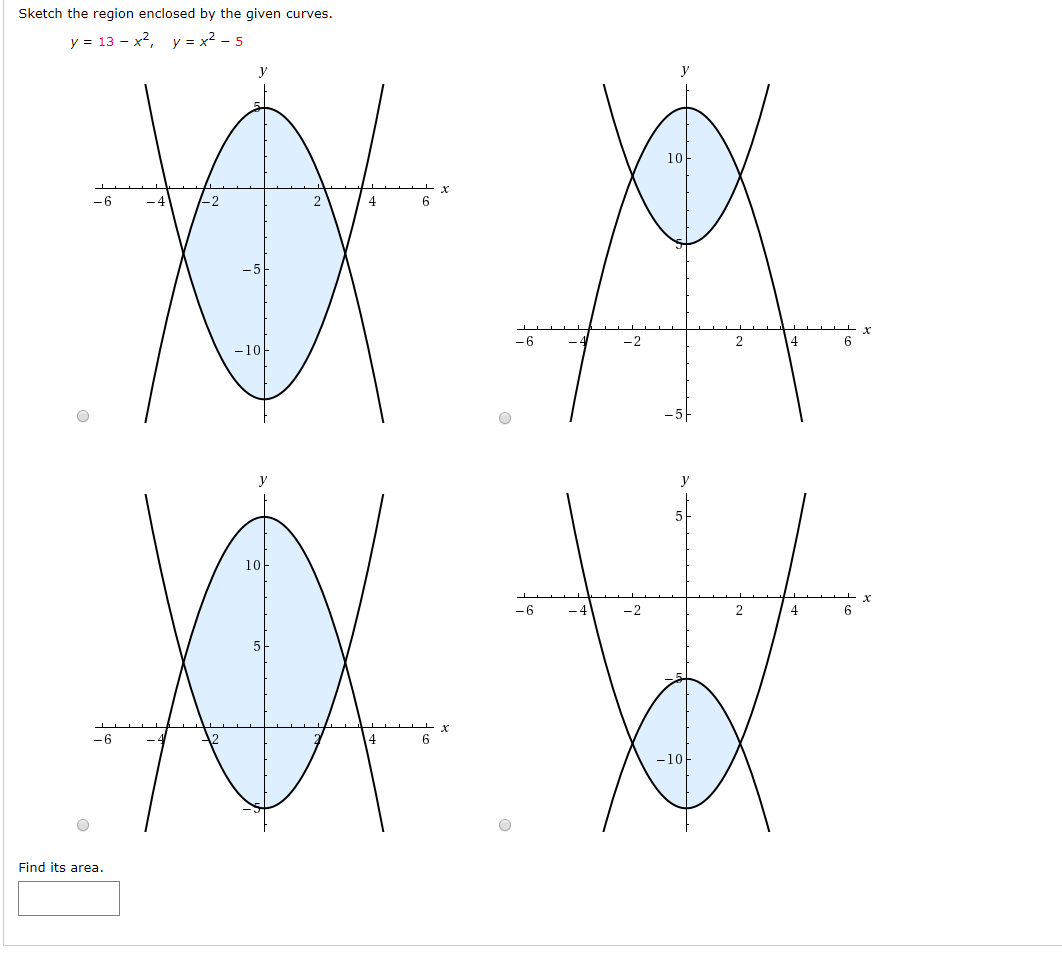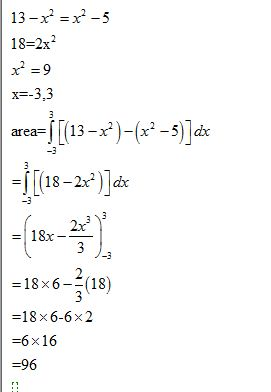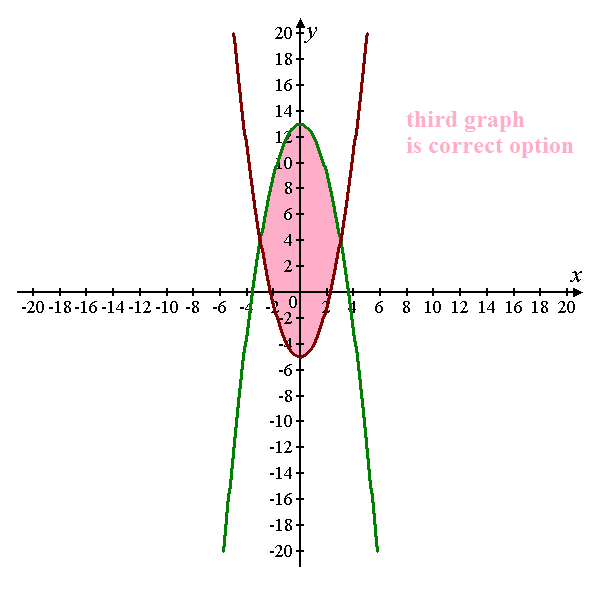#### Earn Coins

Coins can be redeemed for fabulous gifts.

Similar Homework Help Questions
• ### 4. Sketch the region enclosed by the curves y = x, y = 4x, y =...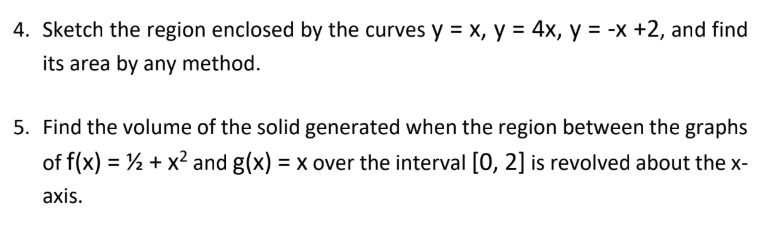4. Sketch the region enclosed by the curves y = x, y = 4x, y = -x +2, and find its area by any method. 5. Find the volume of the solid generated when the region between the graphs of f(x) = 1 + x2 and g(x) = x over the interval (0, 2) is revolved about the x- axis.

• ### Sketch the region enclosed by the given curves. y = 8 cos ( x ) ,...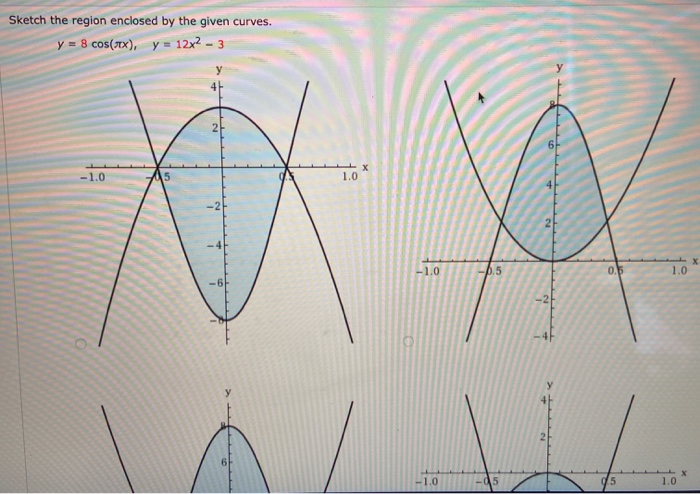Sketch the region enclosed by the given curves. y = 8 cos ( x ) , y 3 - تهر12= 하 5 ا ا / \ م | للللللللللل 1 5 1.0 1.0 -15 Find its area.

• ### Sketch the region enclosed by the curves y = x + 2, y = 16 –...

Sketch the region enclosed by the curves y = x + 2, y = 16 – x2 , x = – 2, and x = 2 on your paper. Find the area of the region. Show all steps mathematically connected.

• ### Sketch the region enclosed by the given curves. Then, find its area by integrating with respect...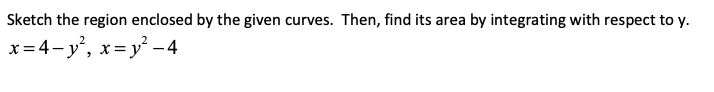Sketch the region enclosed by the given curves. Then, find its area by integrating with respect to y. x=4-y’, x= y² - 4

• ### help Sketch the region enclosed by the given curves. Decide whether to integrate with respect to...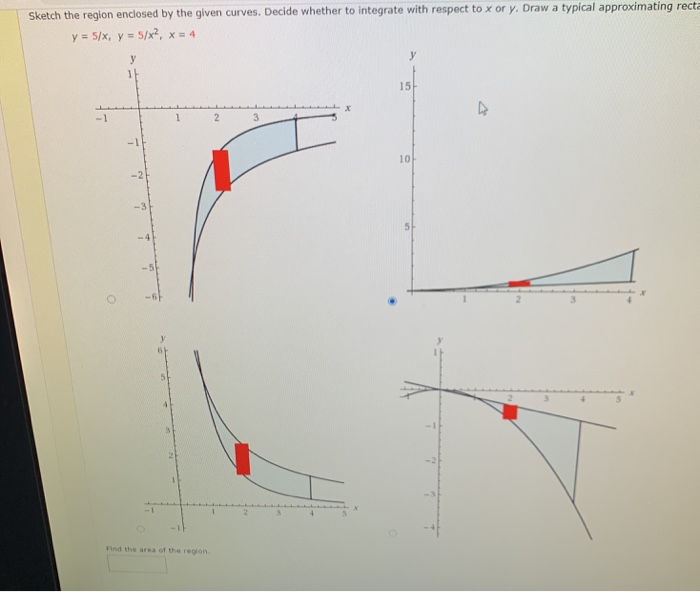help Sketch the region enclosed by the given curves. Decide whether to integrate with respect to x or y. Draw a typical approximating recta y = 5/x, y = 5/x?, x = 4 у у 11 15 - 1 2 3 -1 10 -2 5 4 Find the area of the region

• ### 5. Sketch the region enclosed by the curves y = (x – 2)2 and y =...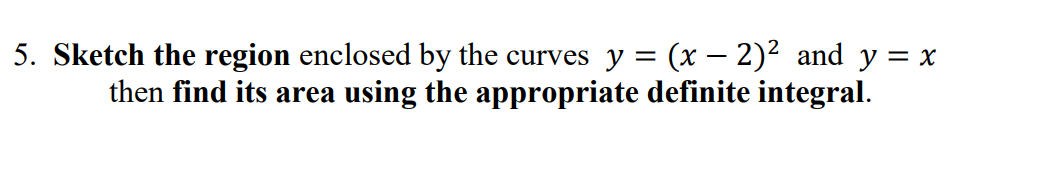5. Sketch the region enclosed by the curves y = (x – 2)2 and y = x then find its area using the appropriate definite integral.

• ### Sketch the region enclosed by the given curves. Decide whether to integrate with respect to x...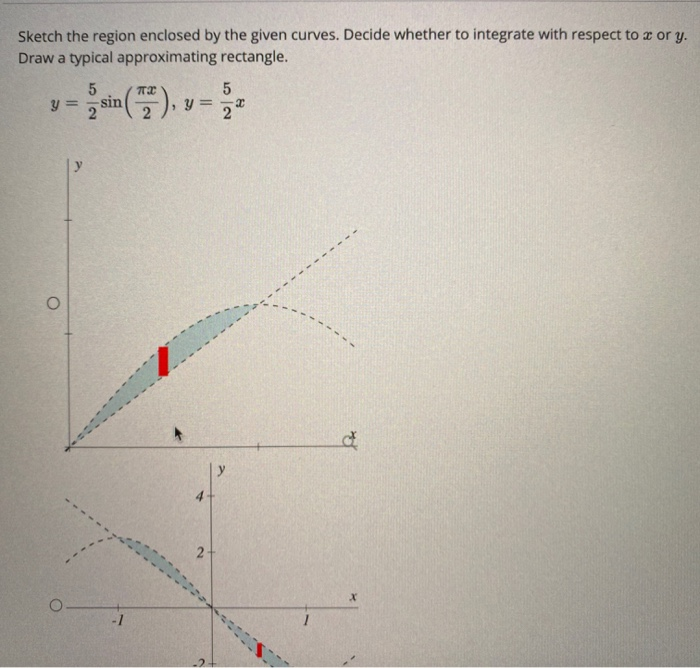Sketch the region enclosed by the given curves. Decide whether to integrate with respect to x or y. Draw a typical approximating rectangle. 5 5 2 22 TE y = - sin y = у O d у 2 X у a y 4 -- 2 х -1 1 ----*====:2: -2 -4+ Q Find the area of the region.

• ### Sketch the region enclosed by the given curves. Decide whether to integrate with respect to x...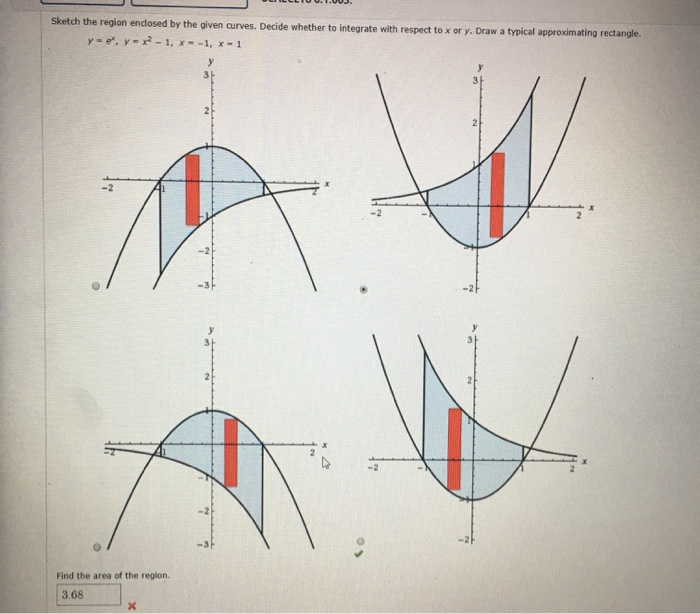Sketch the region enclosed by the given curves. Decide whether to integrate with respect to x or y. Draw a typical approximating rectangle. y e , y=x2-1, x=-1, X-1 Find the area of the region. 3.68

• ### Sketch the region enclosed by the given curves. y - 110x1, y = x2 - 11...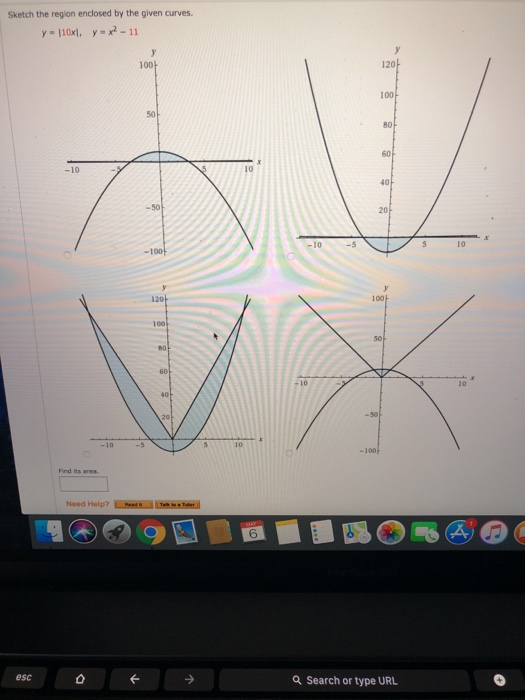Sketch the region enclosed by the given curves. y - 110x1, y = x2 - 11 1001 D510 888 Need Help? The esc Q Search or type URL

• ### Find the area of the region enclosed by the following curves. (10 Puan) y = x2...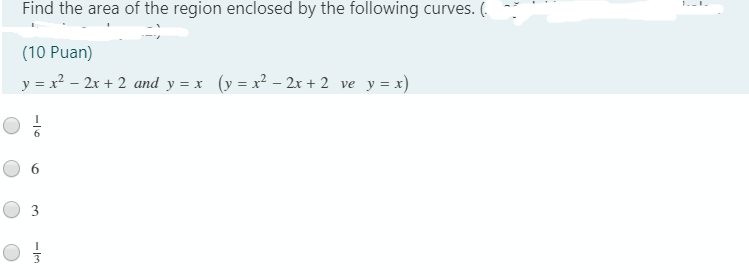Find the area of the region enclosed by the following curves. (10 Puan) y = x2 – 2x + 2 and y = x (y = x2 – 2x + 2 ve y = x) O 1 3